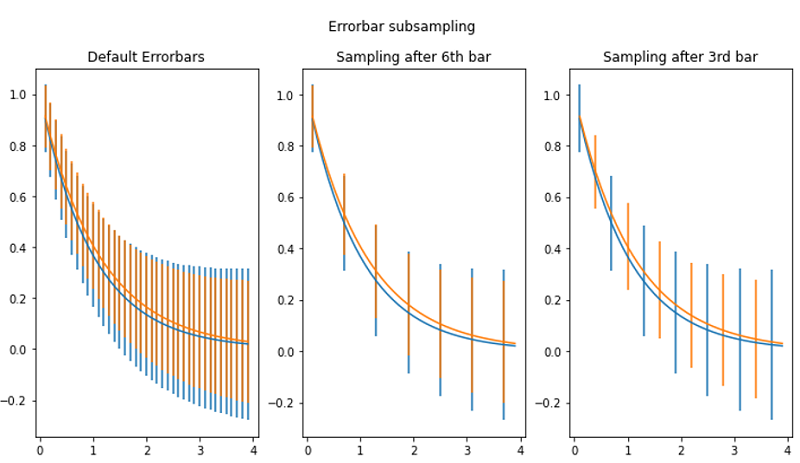# Python | Error bar Sampling using Object Oriented Style in matplotlib

In this tutorial, we are going to learn how to plot error bar and perform sampling using matplotlib in Python?
Submitted by Anuj Singh, on August 17, 2020

In a few cases, we need to plot sampled error bars and, in this article, we are going to present error bar illustration using object-oriented style plotting. The following figure includes the default error bar plot along with sampled error bars.

Illustrations:## Python code for error bar sampling using object-oriented style in matplotlib

```import numpy as np
import matplotlib.pyplot as plt

x = np.arange(0.1, 4, 0.1)
y1 = np.exp(-1.0 * x)
y2 = np.exp(-0.9 * x)

y1err = 0.1 + 0.1 * np.sqrt(x)
y2err = 0.1 + 0.1 * np.sqrt(x/2)

fig, (ax0, ax1, ax2) = plt.subplots(nrows=1, ncols=3, sharex=True,figsize=(12, 6))

ax0.set_title('Default Errorbars')
ax0.errorbar(x, y1, yerr=y1err)
ax0.errorbar(x, y2, yerr=y2err)

ax1.set_title('Sampling after 6th bar')
ax1.errorbar(x, y1, yerr=y1err, errorevery=6)
ax1.errorbar(x, y2, yerr=y2err, errorevery=6)

ax2.set_title('Sampling after 3rd bar')
ax2.errorbar(x, y1, yerr=y1err, errorevery=(0, 6))
ax2.errorbar(x, y2, yerr=y2err, errorevery=(3, 6))

fig.suptitle('Errorbar subsampling')
plt.show()
```

Output:

```Output is as Figure
```